# t-SNE (t-Distributed Stochastic Neighbor Embedding) Algorithm

Whenever we train any machine learning model, the first step is data refining and selecting valuable features from the available dataset. If we consider every feature as a dimension to visualize, we can not imagine more than three dimensions. That’s where we require dimensionality reduction techniques, and t-SNE is one of many such techniques present in the market.

## Key takeaways from this blog

After going through this article, we will be able to understand the following things:

1. What is t-SNE?
2. t-SNE vs. PCA
3. Stepwise working of the t-SNE algorithm
4. What is the concept of similarity?
5. Python implementation of t-SNE
6. Mathematical analysis of the t-SNE algorithm

So let’s start without any further delay and try to find the answer to our first question.

## What is t-SNE?

t-SNE is a non-linear dimensionality reduction algorithm used for exploring high-dimensional data. It stands for t-distributed Stochastic Neighbor Embedding, where t represents “t-distribution”. It is stochastic as stepwise mathematical methods are used in t-SNE. It includes neighbors as while calculating the similarity, we consider the number of neighbors each point has and the distance between them.

### What is the curse of dimensionality and dimensionality reduction?

Considering we have multiple feature dimensions, how will we visualize them? It is easy to imagine 2 and 3 dimensions, but we don’t have any technique beyond that. This is also known as the Curse of Dimensionality. Also, more features lead to an increase in training time. So algorithms like PCA, t-SNE and some autoencoders help us reduce the number of dimensions by keeping the overall information the same as what original features were carrying. This process of reducing the number of dimensions is known as dimensionality reduction.

## t-SNE vs. PCA

One common question that might be bothering us is that PCA is a prevalent technique we commonly use, so why do we even need t-SNE?
The most significant difference is that PCA is a linear dimensionality reduction technique. At the same time, t-SNE is a non-linear dimensionality reduction method, and it has an advantage in this case. Similar data points must be represented close together (It is said that points with similar characteristics are together, whether in a higher dimension or a lower dimension), which is not what linear dimensionality reduction algorithms do. If we remember our PCA discussion, our aim was never to keep the neighbors intact but to keep the maximum variance dimensions for more information. And also, t-SNE is way more sophisticated as it was coined in 2008, and PCA is an old algorithm from 1933. So let’s understand how it works.

## Stepwise working of the t-SNE algorithm

We will see an example for dimensional reduction using t-SNE, from 2d to 1d. As shown in the figure below, we consider 3 clusters (cluster is defined as a set of points having similar characteristics) of 2D points.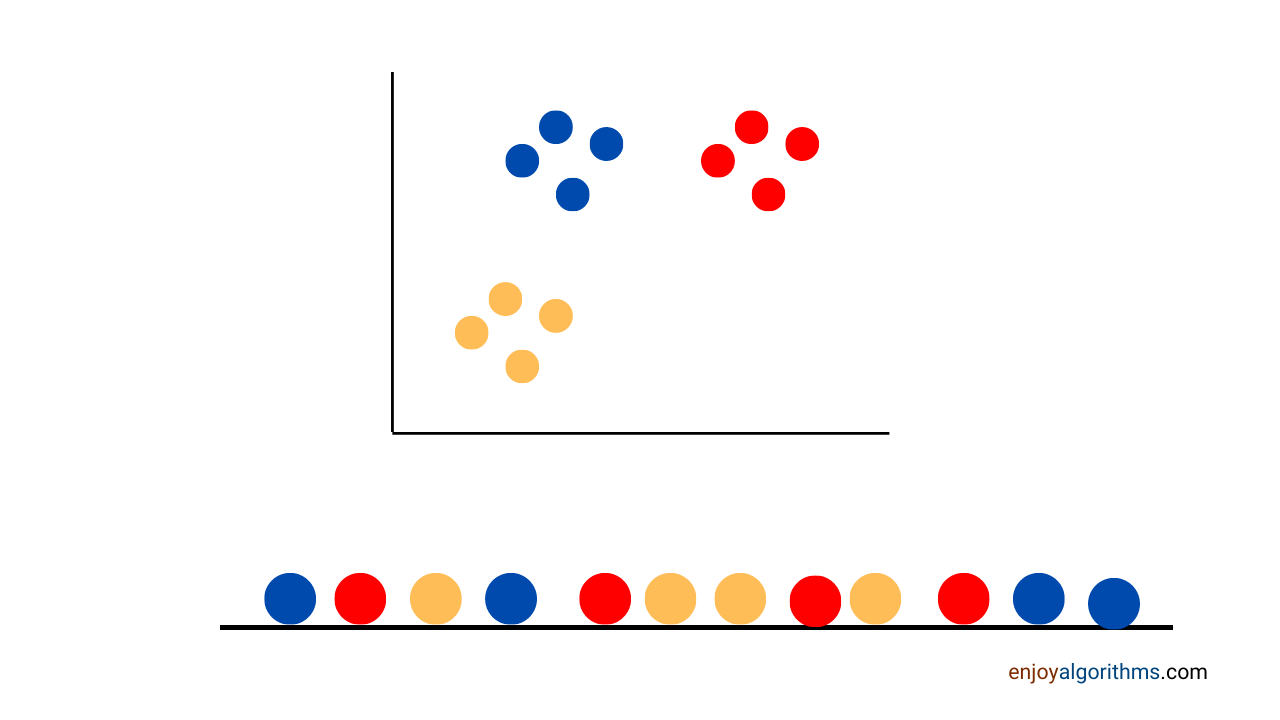Dimensional reduction is not made simply like projecting data on a single axis and getting our result, which leads to a loss of information. For example, lets take three points : (23,31), (47,31.5), (24,32) and there corresponding projection on the y-axis : (0,31), (0.31.5), (0,32). These three points in 1D now appear near each other, which is not the case in 2D as clusters are far apart. t-SNE avoids such a situation and ensures that the corresponding relation of points, which is evident in the higher dimension, is preserved in the lower dimensions.

Let us break it down into steps to simplify the process and visualize it thoroughly.

### Step 1: Initialization of Points

We plot all the points randomly on a 1d line shown in the image above. Next, we have to move them step-by-step such that points of similar characteristics gather together, whereas points of different characteristics move far apart. These points will be moved based on the similarity that we calculate. So let’s see

### Step 2: Concept of Similarity and its use

The similarity is defined as a probability distribution. The probability distribution is defined for two points, A to B, as a conditional probability that A will pick B as its neighbor.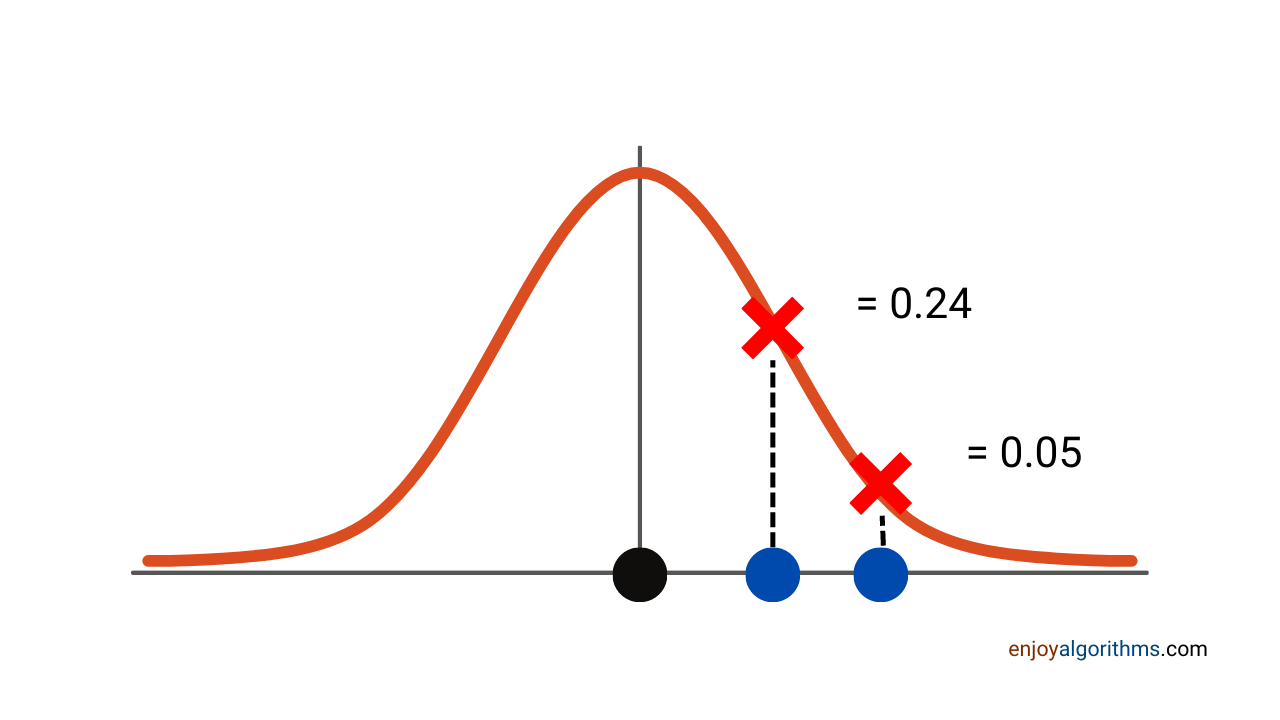So what we do here is choose a point and then build a curve with that point as the center (the black dot in the above figure). It is a 2d plot with each point represented on the x-axis. The height of each point on the curve is based on similarity, i.e., the more similar the point, the closer they are to the center and the more the value of the y axis. Here 0.24 is closure in similarity, so it is near the central point.

Now we need to handle the problem where the sets of points are the same(the best example of such a case is 4 points forming a square but of different lengths). Here, t-SNE perplexity varies as similarity has different values in such cases.

### Step 3: t-SNE perplexity

Scale the unscaled similarities so that they add up to 1. This means that different plots will have different perplexities. Perplexity tells the density of points relative to a particular point. If 4 points of similar characteristics are clustered together, they will have higher perplexity than those not clustered together. Now, points with less density around them have flatter normal curves compared to curves with more density. In the following case, purple points are sparse.

###Step 4: Normalizing Perplexities

But now what happens is suppose we consider 4 points for denser plots and 4 points for less dense plots, they have a similar ratio of distance (dense has values double that of less dense). These 2 sets of 4 points are the same if seen separately and deserve the same treatment, but that will not be the case as y values differ, i.e., similarity differs.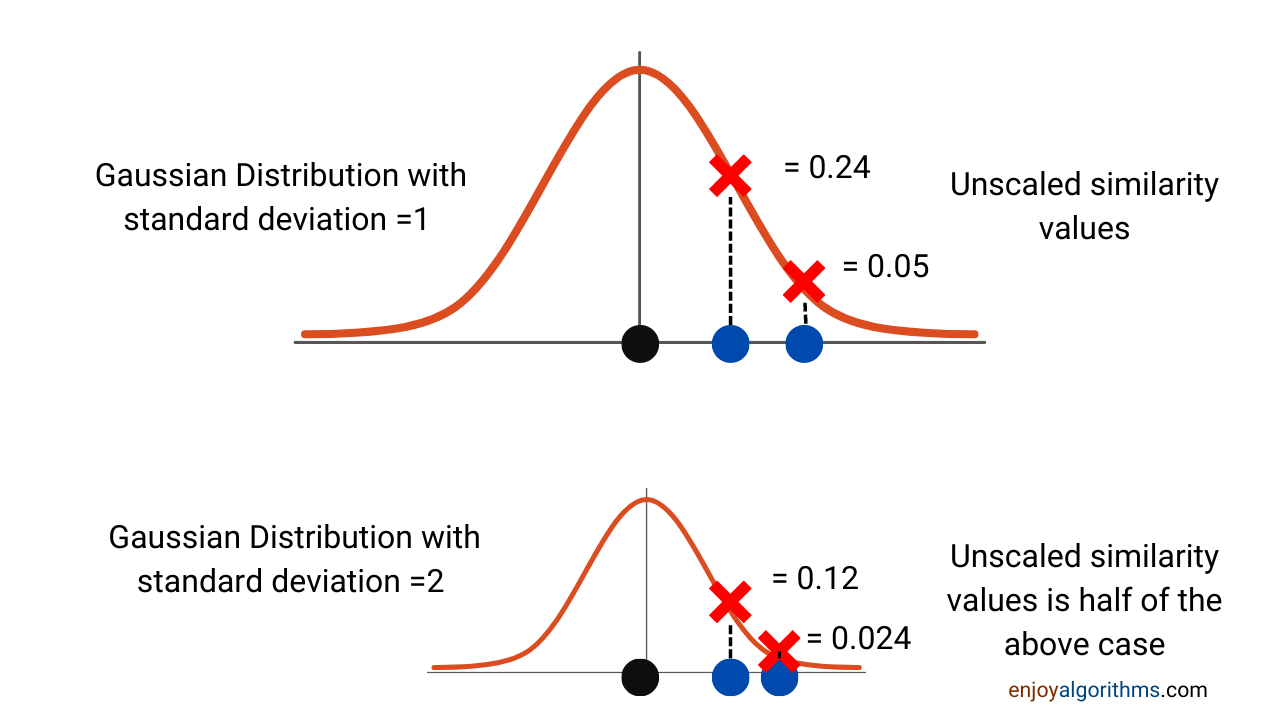So we average values to make the overall similarity sum of one for each graph. This is done by dividing similarity by overall similarity scores obtained in that graph. (Score)/(Sum of all scores) = Scaled Score

• (0.24)/(0.24 + 0.05) = 0.82 and (0.05)/(0.24 + 0.05) = 0.18
• (0.12)/(0.12 + 0.024) = 0.82 and (0.024)/(0.12 + 0.024) = 0.18

Please note that the similarity score of point A with respect to point B will not be the same as point B with respect to point A. This is because the density of points around these two points might vary, and we might have two different curves for them; hence the value can differ. So we consider average values in these cases. So here below, we see that the blue point graph will be different from the yellow point.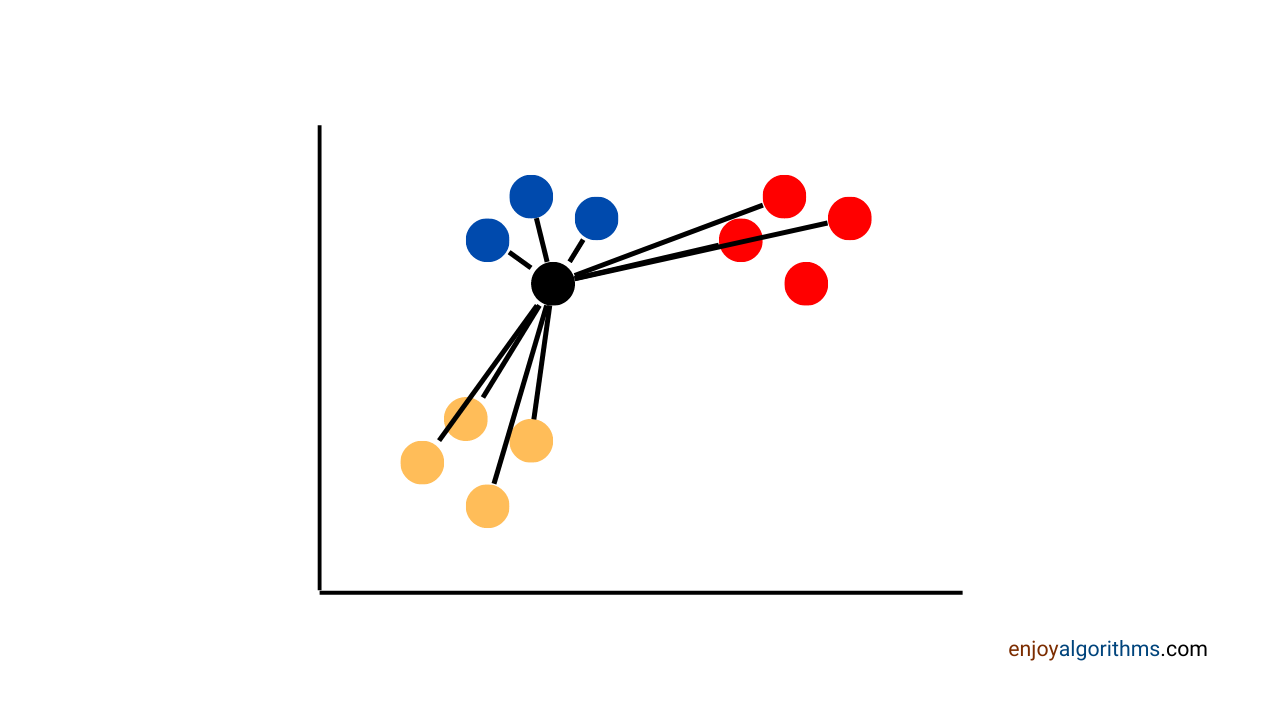### Step 5: Making Similarity Matrix

We end up with a similarity matrix where we can see the points with high similarity scores, which means they belong to the same cluster. Please note that, as we can see matrix defines the similarity of a point to itself(in dark red), but it does not make any sense to do that, so t-SNE defines it as 0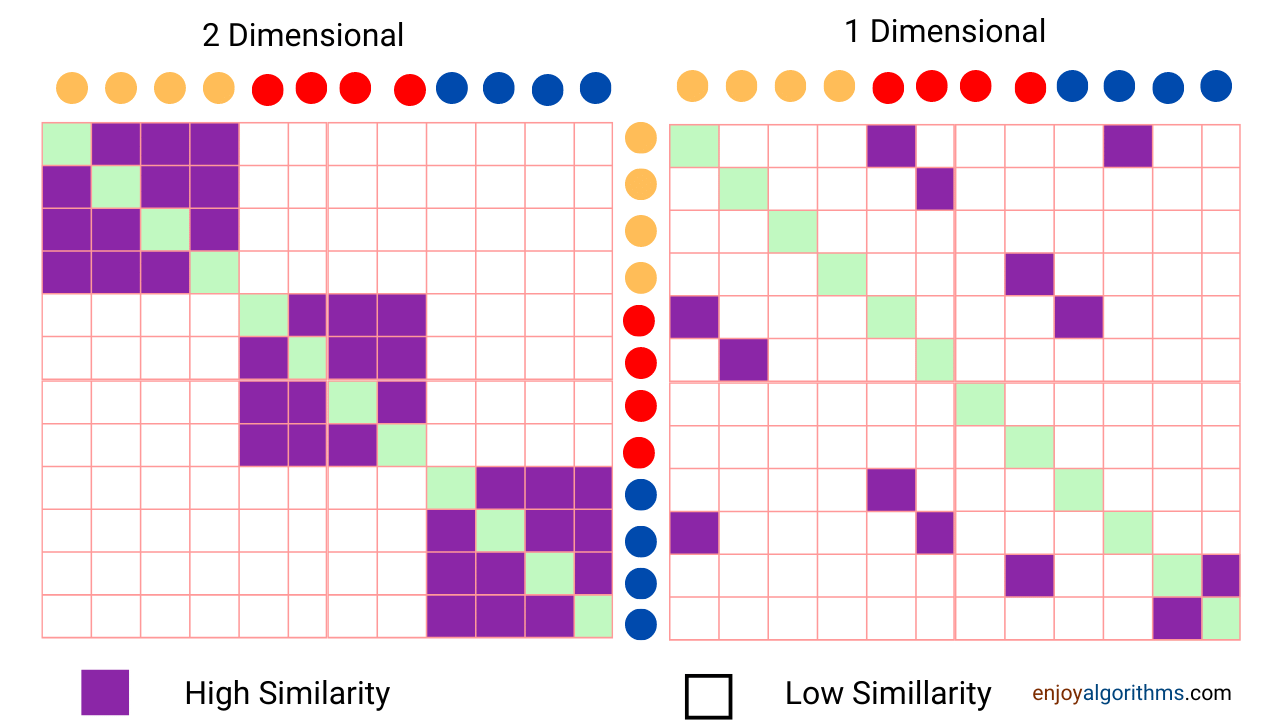We plot the similarity matrix for our dimensionally reduced, i.e., linear mappings in this case, and try to get a matrix similar to the original similarity matrix.

Using this similarity matrix, we move the points on the x-axis to the left or right and adjust them so that the similarity matrix of 1D becomes the same as 2D.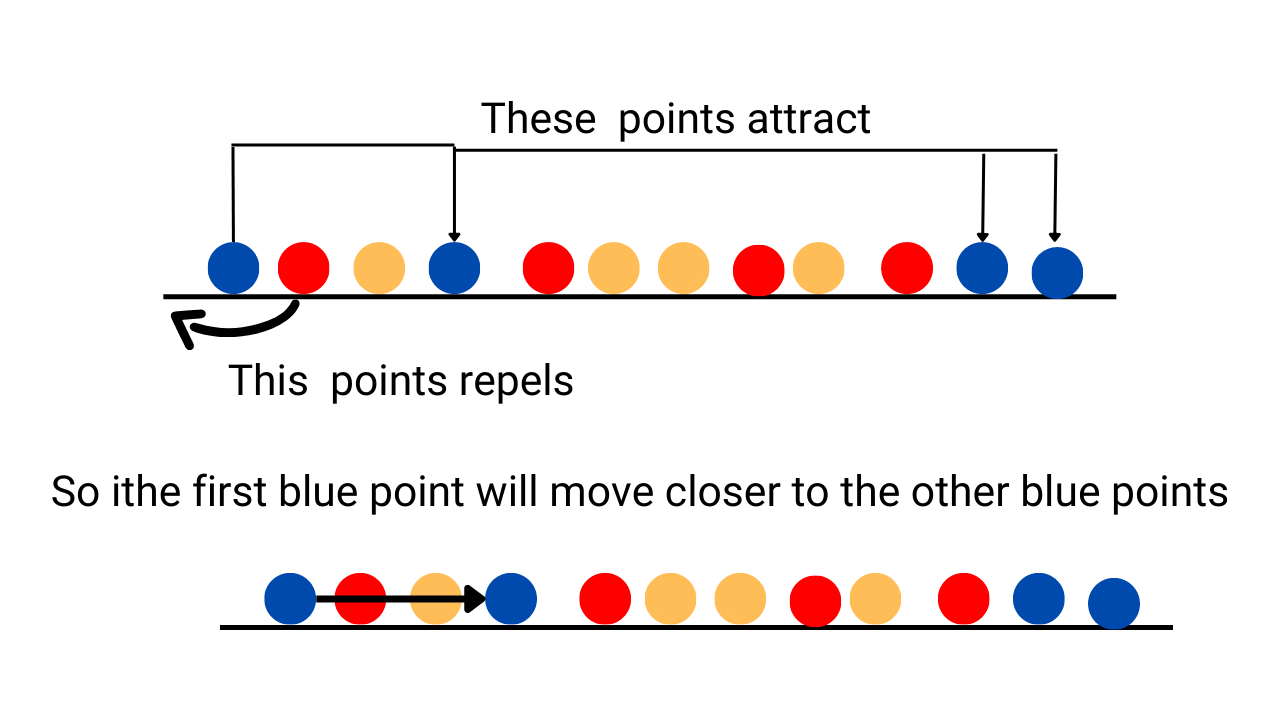Based on the matrix, we get from similarity scores as we see that the orange point moves towards the right, i.e., even though we have attraction and domination but which will dominate is decided as discussed before. So finally, we get the points as we should see, and similar characteristics points occur together.1. Handles Non-linear Data, unlike PCA, which takes linear data
2. Preserves Local Structure: In PCA, we don’t maintain local structure after dimension reduction as it cares about variance. But here, the local structure is maintained i.e., points nearby in original dimensions will also be nearby after dimension reduction. So it helps us to capture the essence of the data

1. Computational Complexity: t-SNE involves complex calculations as it calculates the pairwise conditional probability for each point. Due to this, it takes more time as the number of data points increases.
2. Non-Deterministic: Sometimes, we may get different results with the same hyper-parameters. This means that even though code and data points are the same in each iteration, we may get different results.

Too much theory, let’s jump toward the implementation of t-SNE using python.

## Python implementation of t-SNE

### Step 1: Necessary Libraries to be imported

• pandas: Used for creating a dataframe to read the data file
• matplotlib: Creating scattered data points
• from sklearn.manifold import t-SNE: For training standard t-SNE model from sklearn.
• from sklearn.preprocessing import StandardScaler: To normalize data so that some features are not preferred due to having significant values
``````# Importing Necessary Modules.
import numpy as np
import pandas as pd
import matplotlib.pyplot as plt
from sklearn.manifold import TSNE
from sklearn.preprocessing import StandardScaler
import seaborn as sn``````

The dataset used for this project is the MNIST dataset. The MNIST dataset is an acronym that stands for the Modified National Institute of Standards and Technology dataset. It contains 60,000 small square 28×28 pixel grayscale images of handwritten single digits between 0 and 9. There are 60000 data points with 784 (28 * 28) features. There is also a label that we remove from features.

``````# Reading the data using pandas
labels = df['label']
# Drop the label feature and store the pixel data in dataframe
data = df.drop("label", axis = 1)``````

The dataframe can be seen below, which is seen using the head function of pandas.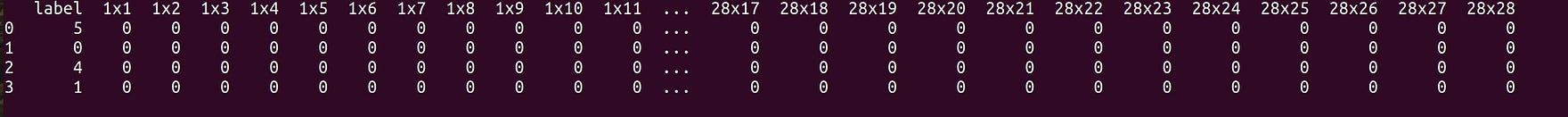### Step 3: Normalizing data

Here we normalize data to prevent it from giving weights to particular values. After normalization, it ensures that even if some values were more significant initially, it would not affect our model negatively. For normalizing, we use the standard scaler function of sklearn. Also, we choose 1000 data points here to show the effect of our model.

``````standardized_data = StandardScaler().fit_transform(data)
print(standardized_data.shape)
data_1000 = standardized_data[0:1000, :]
labels_1000 = labels[0:1000]``````

### Step 4: Training of the t-SNE model

Here we train the model using the standard option of sklearn and using the top 1000 data points.

``````model = TSNE(n_components = 2, random_state = 0)
# configuring the parameters
# the number of components = 2
# default perplexity = 30
# default learning rate = 200
# default Maximum number of iterations
# for the optimization = 1000
tsne_data = model.fit_transform(data_1000)``````

### Step 5: Plotting of data

Here finally, we draw a scatter plot using matplotlib in 2D as our t-sne model is in 2D. So our features get reduced from 784 to 2. We see here that similar points are grouped.

``````tsne_data = np.vstack((tsne_data.T, labels_1000)).T
tsne_df = pd.DataFrame(data = tsne_data,
columns =("Dim_1", "Dim_2", "label"))

# Ploting the result of tsne
sn.FacetGrid(tsne_df, hue ="label", size = 6).map(

plt.show()``````

The results of the plot are shown below. The labels are numbers from 0 to 9, and we see a good deal of these clusters together.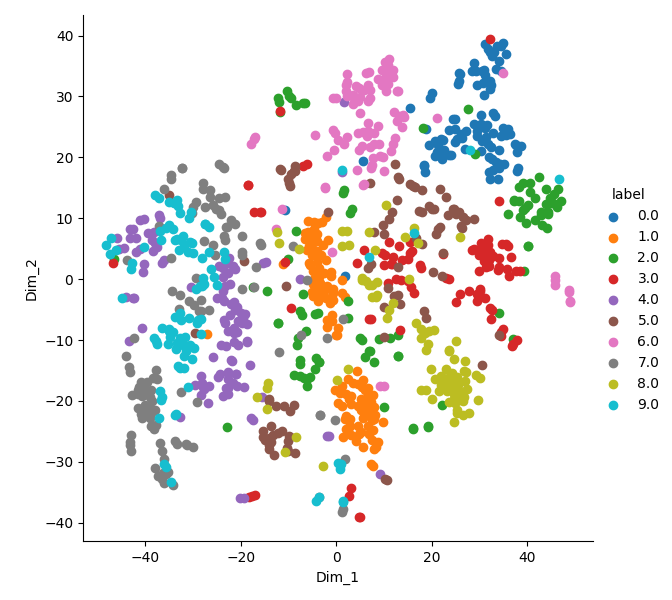## Mathematical Analysis of t-SNE Algorithm (Bonus)

Now we will see the mathematical theory of how the t-SNE algorithm works. The formula for normal distribution.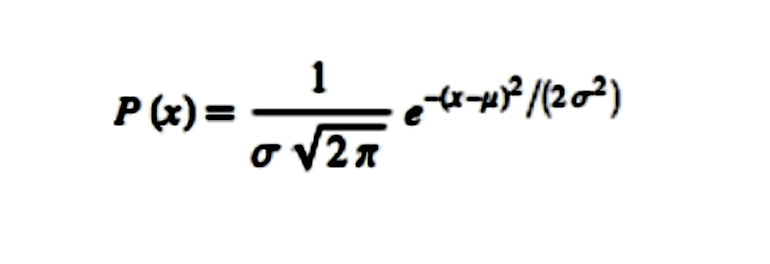The above formula is with respect to mean. But in t-SNE, we calculate with respect to every point and not mean. So if we replace the mean with a point, we get the following formula.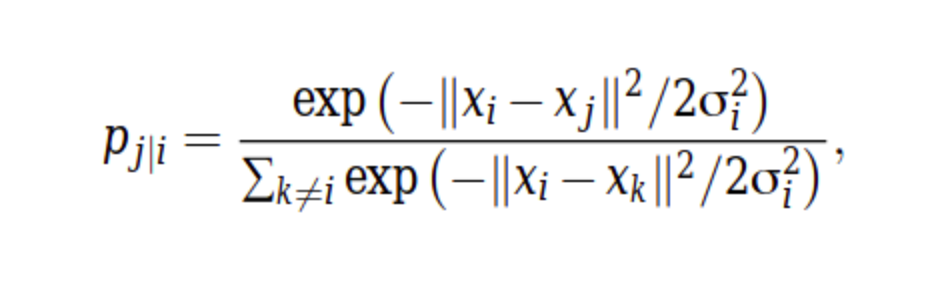We need to define a matrix with the dimension of nsamples * ncomponents and randomly fill the values. Like in our case, we have 12 samples, and the number of components is 1(final dimensions are considered here). So, in this case, 12*1, we fill it with random values. We will use the following formula.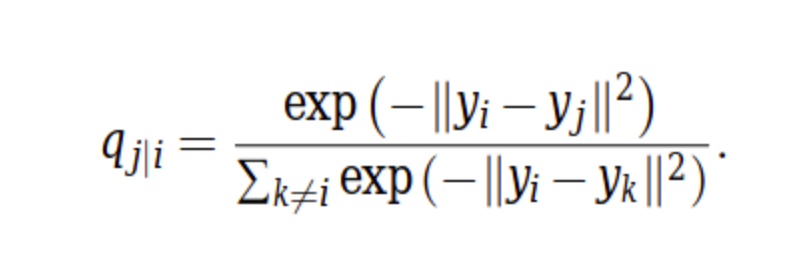We have to make sure that these probability distributions with respect to every point are the same as possible. For this, we use the Kullback-Leiber Divergence method. This KL method is defined as how one probability distribution differs from another. So lower the value, the more the identical distributions are. For example, 0 means that the two distributions are precisely the same.

So we now need to minimize the KL divergence values. Hence our cost function is based on this.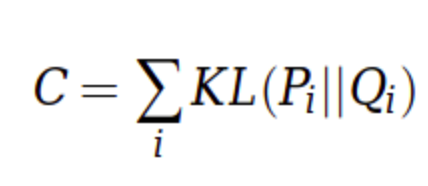The above cost function is minimized to give us directions in which points proceed, i.e., to left or right on a single number line. That’s all for this topic.

## Possible Interview Questions on the t-SNE Algorithm

t-SNE is quite famous among data scientists. If we are applying for the data scientist or machine learning roles, interviewers can ask the following questions on this topic:

1. What is t-SNE, and how is it different from PCA?
2. Mention some advantages of t-SNE over PCA.
3. What is perplexity, and why do we need to normalize it?
4. Why do we need to normalize the data before applying t-SNE?
5. What is the need for a dimensionality reduction technique and the curse of dimensionality?

## Conclusion

In this article, we discussed another dimensionality reduction technique used to select the valuable features from the available dataset. We discussed the stepwise process of the t-SNE algorithm on a dummy dataset and implemented it on the MNIST dataset using python. Later we discussed the inlined mathematical details of this topic. We hope you enjoyed the article.